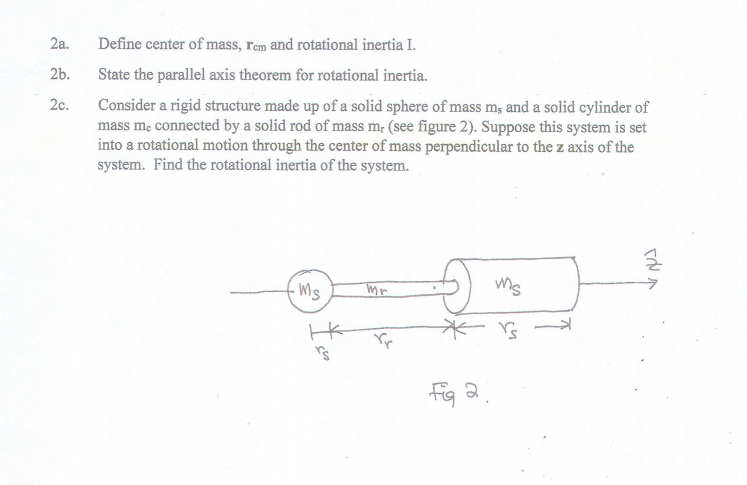# 2a.2b.2c.Define center of mass, rem and rotational inertia IState the parallel axis theorem for rotational inertia.Consider a rigid structure made up of a solid sphere of mass ms and a solid cylinder ofmass me connected by a solid rod of mass mr (see figure 2). Suppose this system is setinto a rotational motion through the center of mass perpendicular to the z axis of thesystem. Find the rotational inertia of the system.ms码a.

Question
49 viewshelp_outlineImage Transcriptionclose2a. 2b. 2c. Define center of mass, rem and rotational inertia I State the parallel axis theorem for rotational inertia. Consider a rigid structure made up of a solid sphere of mass ms and a solid cylinder of mass me connected by a solid rod of mass mr (see figure 2). Suppose this system is set into a rotational motion through the center of mass perpendicular to the z axis of the system. Find the rotational inertia of the system. ms 码a. fullscreen
check_circle

Step 1

(2a)

Center of mass:

Center of mass of a system is defined as a single point at which the whole mass of the system is imagined to be concentrated and all the applied forces acts at that point.

Center of mass of body depends on its geometrical shape. If a system is symmetrical and uniform composition, the center of mass will be located at its geometrical center.

Step 2

For example:

• Center of mass of square is at the point of intersection of its diagonals.
• Center of mass of a rigid bar is the middle point.

Write the expression for center of mass of the system.

Step 3

Rotational inertia:

Rotational inertia of a rigid body is a quantity that determines the torque needed for a desired angular acceleration about a rotational ax...

### Want to see the full answer?

See Solution

#### Want to see this answer and more?

Solutions are written by subject experts who are available 24/7. Questions are typically answered within 1 hour.*

See Solution
*Response times may vary by subject and question.
Tagged in

### Centre of Mass and Centre of Gravity